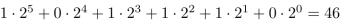# Base or Radix of a number

The base or radix of a number is the maximum number of digits or combination of digits that can be placed in any position.

For example, a number in base-5 can only take digits from 0 to 4 (5 combinations in total). The number 20124 is a good example of a base-5 number. The number 6352 cannot be a base-5 number since it contains the digits 6 and 5.

The decimal system (base-10) is our common way to express numbers. Each digit can be a number from 0 to 9.

For bases greater than 10, as for the hexadecimal system (base-16), there are not enough symbols to express each digit which can be from 0 to 15. We then use capital letters from A to F to represent digits from 10 to 16. For example, a typical hexadecimal number is 4DB1.

For binary numbers (base-2), there are only two allowed symbols: 0 and 1. 10001110 can be a binary number, but 102010 cannot because it contains a 2

## Conversion from base-N numbers to decimal

Each position occupied by a digit corresponds to a power of the base, exactly as for the decimal numbers, whose positions are multiples of powers of ten.

For example, the base-5 number 234 has three digits, each one multiplying a power of 5 as follows:

• 4 times 5^0 = 4
• 3 times 5^1 = 15
• 2 times 5^2 = 50
So the number 234 in base-5 corresponds to the decimal 4+15+50 = 69

The binary 101110 can be converted to decimal by computing:## Conversion from decimal numbers to base-N

The succesive divisions is a well-known method to convert from decimal to any other base. The procedure includes long divisions to get the partial quotients and remainders. Let's take for example the decimal 2643 and let's convert it to hexadecimal.
The first division by 16 produces an integer quotient of 2643/16 = 165 and a remainder of 2643 - 16*165 = 3.
The quotient is now divided again by 16 to get a new (integer) quotient of 165/16 = 10 and a remainder of 165 - 16*10 = 5. This quotient cannot be further divided by 16 so we collect the remainders and the last quotient from last to first to get the numbers 10,5,3 which will be represented in hexadecimal as A53